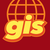# Rounding in label arcade expressions

238
1
10-14-2021 10:42 AMby
New Contributor III

I've run into a small issue in my application and am wondering if someone has already solved it. We're currently using feature reduction clusters and displaying labels on those clusters using an arcade expression.

The expression looks like

``````      labelExpressionInfo: {
expression: `
var count = \$feature.cluster_count*\$feature.cluster_avg_totalAssets
Text(count, '#,### ')
`
}``````

We are trying to achieve rounding within the expression. I know that I can write custom code to handle conditions of the count, but I'm wondering if there is a better way to achieve the visualization shown below where numbers above 1000 round to the nearest hundred and numbers above 100,000 round to the nearest thousand and numbers above 1000000 round to the nearest million.

Is the best path here just to write code to handle each situation?

https://developers.arcgis.com/javascript/latest/sample-code/featurereduction-cluster-filter/

1 Solution

Accepted Solutionsby
New Contributor III

FWIW - and for anyone who comes across it in the future - I did something like this. Not sure if it is the best way ... but it is a way.

``````var count = \$feature.cluster_count*\$feature.cluster_avg_totalAssets

var rounded = When(
count >= 1000000, (Round(count, 0) / 1000000) + "M",
count >= 10000, (Round(count / 1000, 0) * 10000) / 10000 + "K",
count >= 1000, (Round(count / 100, 0) * 100) / 1000 + "K",
Round(count, 0)
)

Text(rounded)
```````by
New Contributor III

FWIW - and for anyone who comes across it in the future - I did something like this. Not sure if it is the best way ... but it is a way.

``````var count = \$feature.cluster_count*\$feature.cluster_avg_totalAssets

var rounded = When(
count >= 1000000, (Round(count, 0) / 1000000) + "M",
count >= 10000, (Round(count / 1000, 0) * 10000) / 10000 + "K",
count >= 1000, (Round(count / 100, 0) * 100) / 1000 + "K",
Round(count, 0)
)

Text(rounded)
```````